Overview

A visual abstract is a great companion to help publicize your study. They are particularly efficient at communicating your main findings at a high level. For more information, the interested reader is directed here.

The purpose of this vignette is to demonstrate how to create customized visual abstracts from scratch, recognizing the shiny application included in abstractr package has certain restraints by design.

Requirements

To get started, you will need to install and load a few packages:

library(ggplot2)
library(gridExtra)
library(emojifont)

A Blank Canvas

We’ll start with a blank canvas. This will provide a useful point of reference for positioning text and graphics of the visual abstract.

Using theme_linedraw() on our canvas allows us to visualize the coordinate system to this end.

canvas <- ggplot(data=data.frame(x=1:10, y=1:10), aes(x=x, y=y))   +
scale_y_continuous(limits=c(1,10), breaks=seq(1,10,0.5)) +
scale_x_continuous(limits=c(1,10), breaks=seq(1,10,0.5)) +
theme_linedraw()

canvas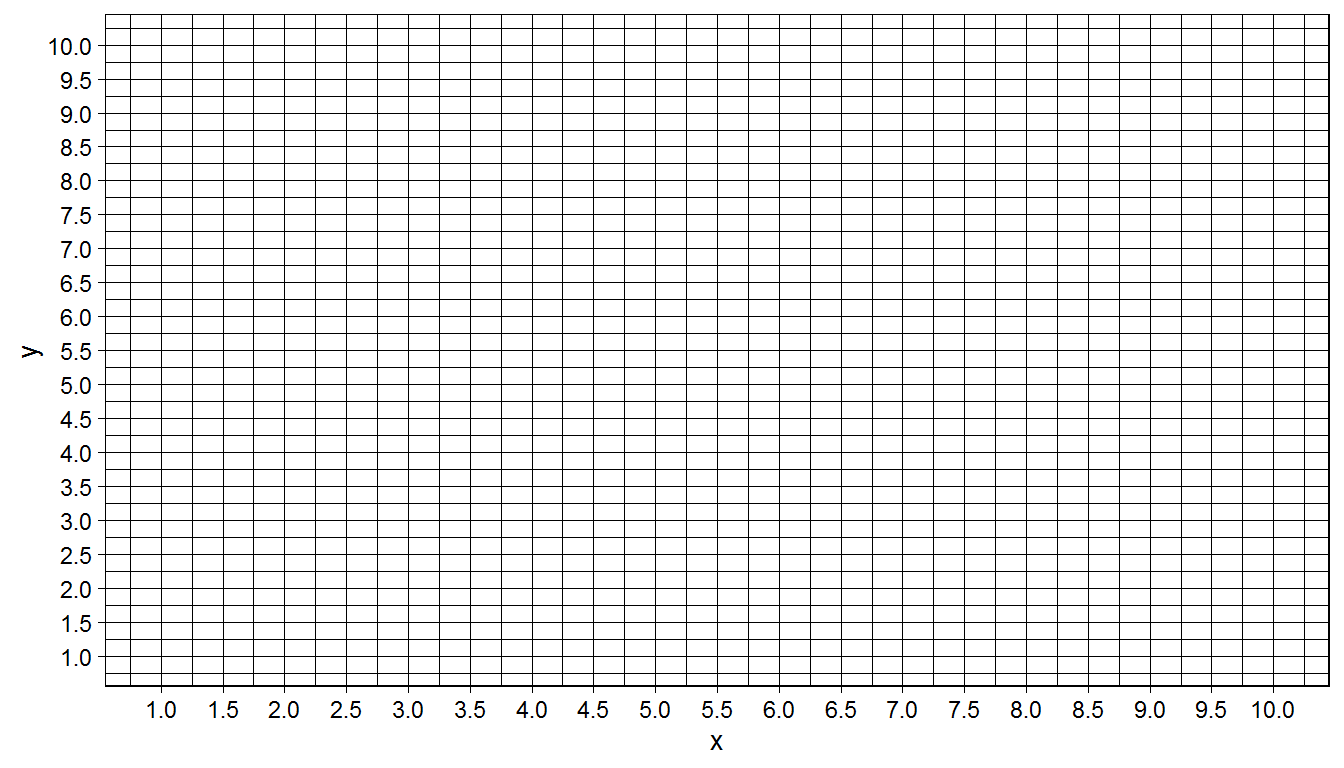Panels

One approach to build a visual abstract is to do so on a panel-by-panel basis. The canvas we created serves as a starting point and we’ll use it to build the first panel.

Let’s remove all existing plot features and color the panel.

panel1 <- canvas +
theme_void() +
theme(panel.background = element_rect(fill = '#DEEBF7', colour = '#DEEBF7'))

panel1This process can be repeated for additional panels and ensures consistency in size of panels so layouts are neat.

For the remaining 2 panels, let’s choose different colors.

panel2 <- canvas +
theme_void() +
theme(panel.background = element_rect(fill = '#9ECAE1', colour = '#9ECAE1'))

panel3 <- canvas +
theme_void() +
theme(panel.background = element_rect(fill = '#3182BD', colour = '#3182BD'))

Layouts

Arranging your panels into a coherent visual abstract is accomplished by grid.arrange().

Using this function, you can specify any configuration you desire for your individual panels. Since we have 3 panels to work with, I can arrange them in columns like so:

grid.arrange(panel1,panel2,panel3, ncol=3)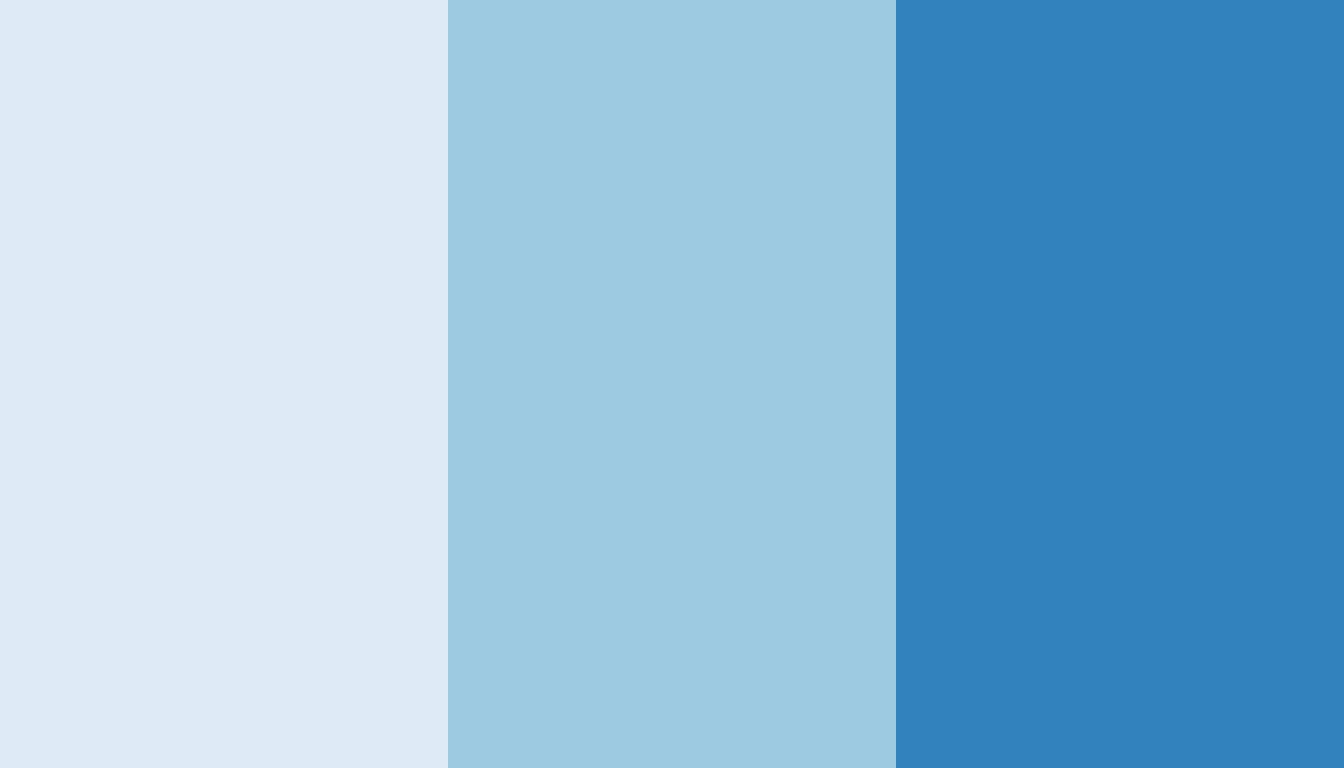The shiny app included in the package is restricted to a column-based layout. If you wish for an alternate layout, you can specify it (or combinations) using grid.arrange(). For example, for a row based layout:

grid.arrange(panel1,panel2,panel3, nrow=3)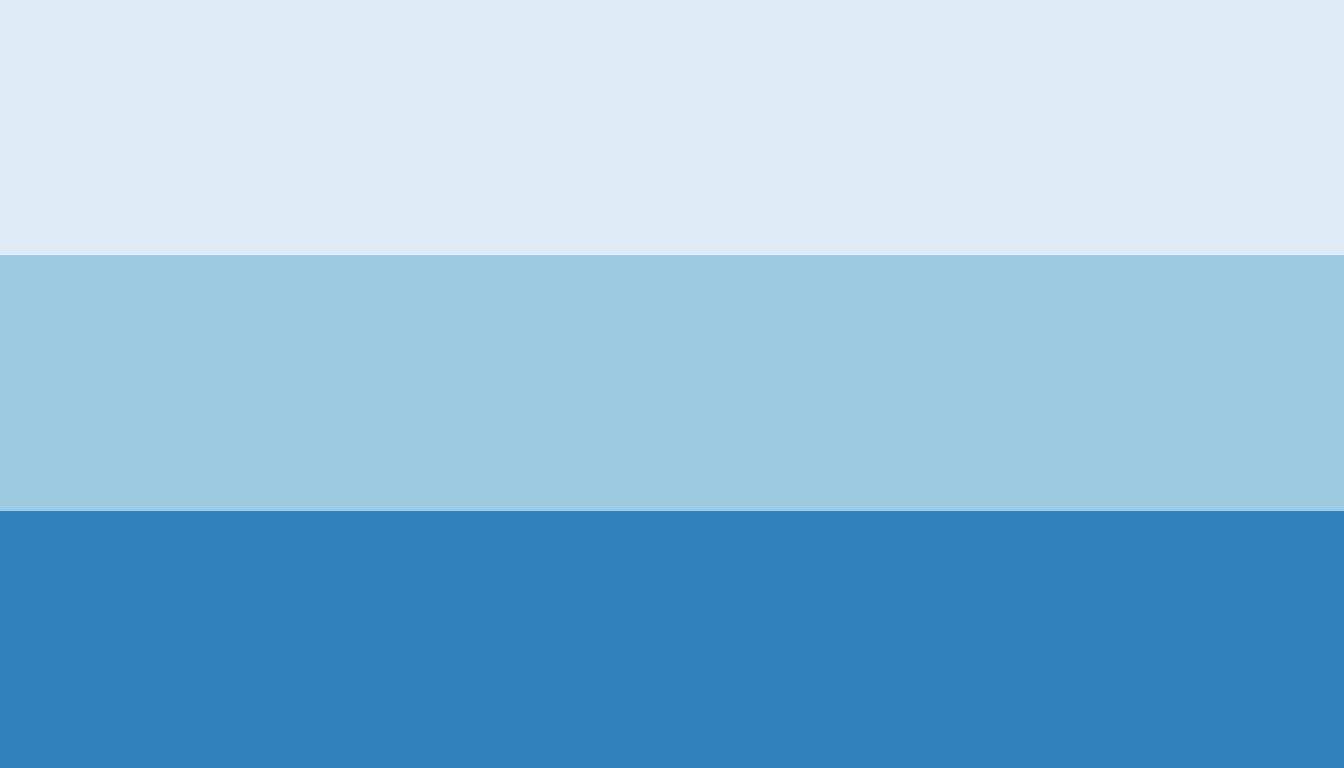Content

Now that we have some panels and a layout in mind, let’s start adding content to individual panels.

Text

Text can be added to a panel using the annotate() function.

We can position the text using the x and y coordinates of our canvas. We can also change text attributes like size, fontface and color.

panel1 <- canvas +
theme_void() +
theme(panel.background = element_rect(fill = '#DEEBF7', colour = '#DEEBF7')) +
annotate("text", x=5.5, y=9,   label = "Finding 1",    size=15, fontface=2) +
annotate("text", x=5.5, y=2.5, label = "Statistics 1", size=12, fontface=2) +
annotate("text", x=5.5, y=1.5, label = "Units 1",      size=12, fontface=2)

panel1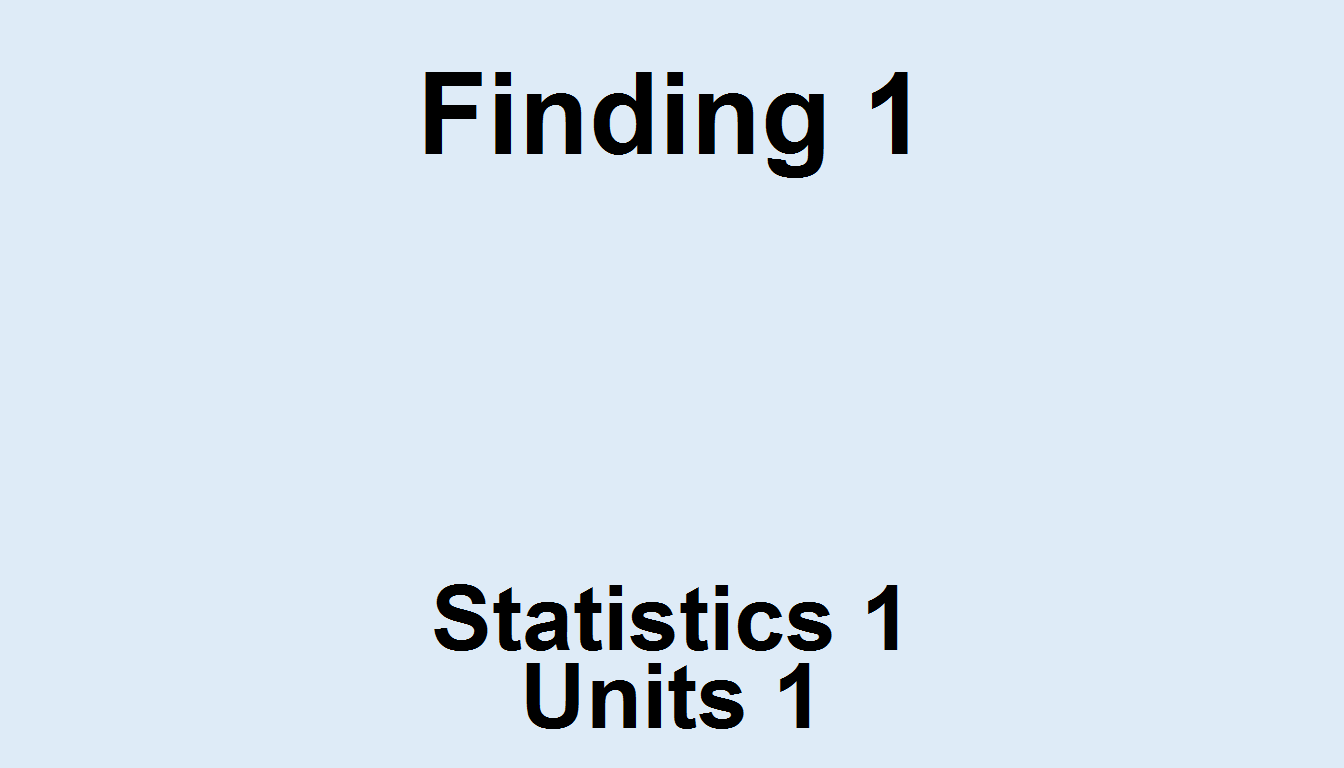Icons

Icons can be added to the panel using the geom_text() function.

Icons are provided by Font Awesome, which are free to use and redistribute. You can view the complete library of icons here.

Let’s use the user-md icon as a demonstration.

panel1 <- canvas +
theme_void() +
theme(panel.background = element_rect(fill = '#DEEBF7', colour = '#DEEBF7')) +
geom_text(family='fontawesome-webfont', label=fontawesome('fa-user-md'),
x=5.5, y=5.5, size=75)Note: If you are using RStudio on Windows, you may have to call a new X11 Window before viewing or the icon might not appear.

panel1 <- canvas +
theme_void() +
theme(panel.background = element_rect(fill = '#DEEBF7', colour = '#DEEBF7')) +
geom_text(family='fontawesome-webfont', label=fontawesome('fa-user-md'),
x=5.5, y=5.5, size=75)

X11()

#panel1

Troubleshooting Positioning

If you want to get an idea of how to set your coordinates, you can simply run a panel without theme_void(). This will render the panel with grid lines.

panel1 <- canvas +
theme(panel.background = element_rect(fill = '#DEEBF7', colour = '#DEEBF7')) +
annotate("text", x=5.5, y=9,   label = "Finding 1",    size=15, fontface=2) +
annotate("text", x=5.5, y=2.5, label = "Statistics 1", size=12, fontface=2) +
annotate("text", x=5.5, y=1.5, label = "Units 1",      size=12, fontface=2)

panel1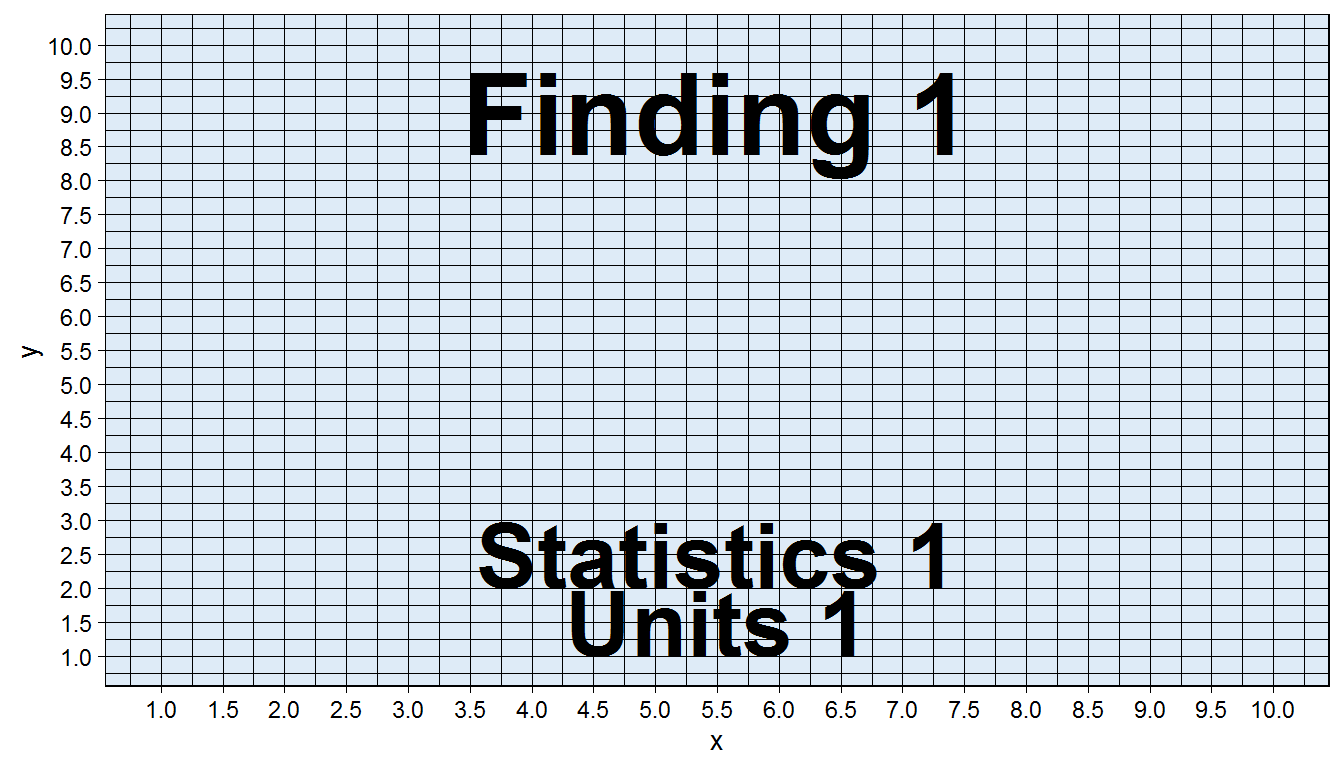You can now clearly see the coordinates specified for the text. This is a useful way for troubleshooting positioning, especially if you change font sizes or want a customized layout. This technique also works for checking the positioning of icons.

Putting It All Together

At this point, you have everything you need to create a visual abstract for your study.

Let’s put things together to see how they work. The code below is a completely self-contained example - feel free to use it as a template.

#Canvas
canvas <- ggplot(data=data.frame(x=1:10, y=1:10), aes(x=x, y=y))   +
scale_y_continuous(limits=c(1,10), breaks=seq(1,10,0.5)) +
scale_x_continuous(limits=c(1,10), breaks=seq(1,10,0.5)) +
theme_linedraw()

#Create individual panels
panel1 <- canvas +
theme_void() +
theme(panel.background = element_rect(fill = '#DEEBF7', colour = '#DEEBF7')) +
geom_text(family='fontawesome-webfont', label=fontawesome('fa-user-md'),
x=5.5, y=5.5, size=75) +
annotate("text", x=5.5, y=9,   label = "Finding 1",    size=13, fontface=2) +
annotate("text", x=5.5, y=2.5, label = "Statistics 1", size=10, fontface=2) +
annotate("text", x=5.5, y=1.5, label = "Units 1",      size=10, fontface=2)

panel2 <- canvas +
theme_void() +
theme(panel.background = element_rect(fill = '#9ECAE1', colour = '#9ECAE1')) +
geom_text(family='fontawesome-webfont', label=fontawesome('fa-ambulance'),
x=5.5, y=5.5, size=75) +
annotate("text", x=5.5, y=9,   label = "Finding 2",    size=13, fontface=2) +
annotate("text", x=5.5, y=2.5, label = "Statistics 2", size=10, fontface=2) +
annotate("text", x=5.5, y=1.5, label = "Units 2",      size=10, fontface=2)

panel3 <- canvas +
theme_void() +
theme(panel.background = element_rect(fill = '#3182BD', colour = '#3182BD')) +
geom_text(family='fontawesome-webfont', label=fontawesome('fa-wheelchair'),
x=5.5, y=5.5, size=75) +
annotate("text", x=5.5, y=9,   label = "Finding 3",    size=13, fontface=2) +
annotate("text", x=5.5, y=2.5, label = "Statistics 3", size=10, fontface=2) +
annotate("text", x=5.5, y=1.5, label = "Units 3",      size=10, fontface=2)

#Arrange individual panels
#grid.arrange(panel1, panel2, panel3, ncol=3)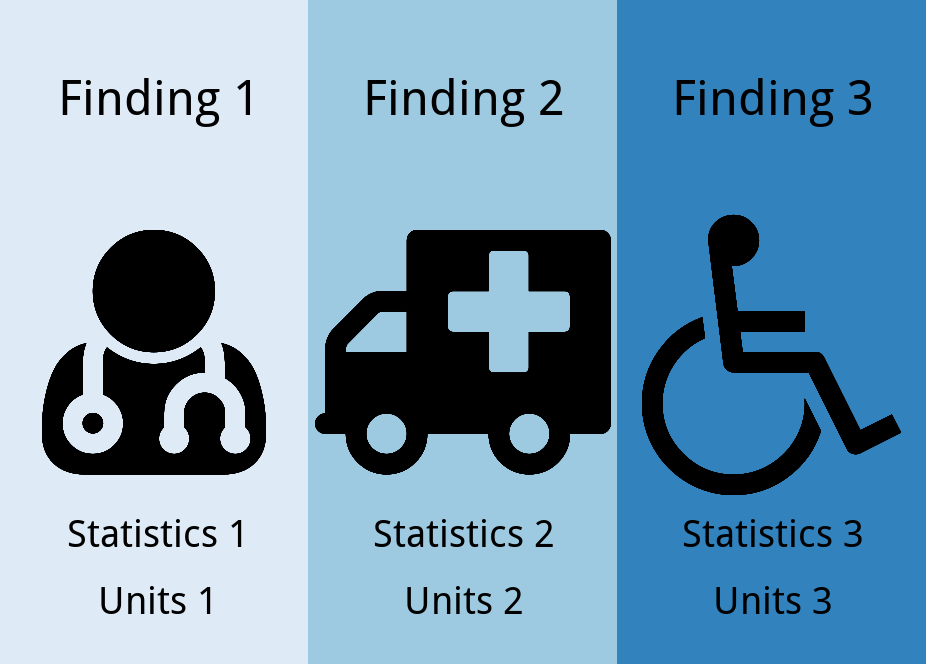If you wish to include headers and footers for your visual abstract, you can create them like you did using the canvas and include them in the layout.